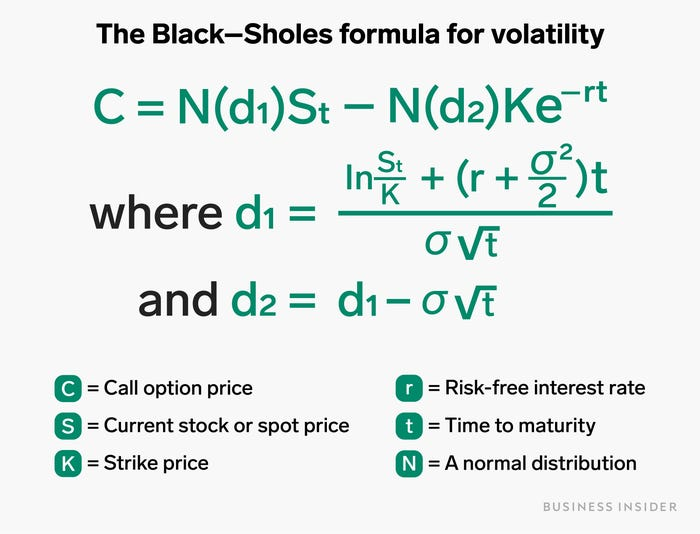# Is “volatility” a risk factor? How to interpret stock price fluctuations | Business Insider JapanStocks with higher volatility tend to move up and down in price.

101cats/Getty Images

• Volatility in investing refers to changes in the price of an asset or market, especially when compared to its normal movements and benchmarks.
• Volatility is often expressed as a percentage. If a stock has a volatility of 10%, it means that the total value of the stock could go up or down by 10%. The higher this number, the greater the fluctuation range of the stock price.
• Volatility and risk are not synonymous, but volatile assets are often viewed as risky because their movements are less predictable.

If you’re thinking about investing, you’ve probably heard the word “volatility” thrown around.

Outside the financial world, volatility means a tendency for rapid and unpredictable change. Definitions in the financial markets are similar, but a little more technical.

The definition of volatility in the market is a statistical measure of how much a stock (or any other asset) can deviate from a particular benchmark or average performance of that stock. Simply put, it is how likely it is that the price of a financial asset, such as a stock, will move suddenly or change significantly.

Not surprisingly, volatility is often viewed as a representative risk factor in investing. Low volatility is safe and likely to produce good results, while high volatility is dangerous and can lead to poor results.

Think of it like riding a bicycle. Safety is never guaranteed when riding a bicycle. It’s normal to stumble a little every once in a while, and usually you don’t really care. However, if the steering wheel is suddenly turned sharply to avoid an obstacle, it becomes difficult to correct the trajectory, which increases the possibility of losing balance and leading to an accident.

There will be winding roads and routes with few turns. Assuming the risks of all routes and mapping out the most dangerous and steep routes is a way to assess and measure volatility.

Additionally, understanding volatility can help you decide when, where and how to invest.

## Volatility type

There are two types of market volatility:

• Historical volatility looking back in time
• Implied volatility predicting the future

Historical volatility (HV), as the name suggests, looks back. It is calculated by observing the movement of a certain stock over a certain period of time in the past and paying attention to how much the price has deviated from the average price of that stock.

Watch out for rising historical volatility as it suggests that something is happening or about to happen in the stock. A decline means normalization and stabilization is progressing.

Future volatility, or implied volatility (IV), is more complicated than that. It predicts the future movement of an asset based on option prices (an option is a contract that gives the buyer the right[but not the obligation]to buy or sell the underlying asset at a specified price on or before a specified date). .

Both HV and IV are expressed as percentages and standard deviations (+ or -). For example, if XYZ stock has a standard deviation of 10%, it means that the total value of the stock could increase or decrease by 10%. In other words, the higher the number, the higher the stock’s volatility.

## Volatility prediction method

Implied volatility is calculated by plugging five indicators into a formula: the option’s market price, the price of the underlying asset (spot price), strike price, life to maturity, and risk-free interest rate (see below). Then work backwards to find the implied volatility, which is an indicator that predicts how much the stock’s value will fluctuate in the future.

For example, take two similarly priced stocks and take put (sell) and call (buy) options on each with the same remaining term. In that case, you might think that the put option price and the call option price are about the same. However, considering the IV changes that expectation. The higher the option price, the higher the implied volatility.

## black-scholes equation

The formula given above for finding IV is called “”. A mathematical model designed to calculate stock market option prices, expressed as:Black-Scholes equation for volatility: C = call option price, S = underlying asset price, K = strike price, r = risk-free interest rate, t = time to maturity, N = normal distribution